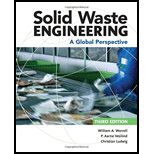# Cellulose is to be burned in a waste-to- energy fadlity. The chemical equation for cellulose C 6 H 1 0 O 5 is the atomic weights of C, H, and O are 12, 1, and 16. respectively. a. Calculate the stoichiometric oxygen necessary for the combustion of cellulose. b. Calculate stoichiometric air.### Solid Waste Engineering

3rd Edition
Worrell + 1 other
Publisher: Cengage Learning,
ISBN: 9781305635203

#### Solutions

Chapter
Section### Solid Waste Engineering

3rd Edition
Worrell + 1 other
Publisher: Cengage Learning,
ISBN: 9781305635203
Chapter 7, Problem 7.10P
Textbook Problem
1102 views

## Cellulose is to be burned in a waste-to- energy fadlity. The chemical equation for cellulose C6 H10O5is the atomic weights of C, H, and O are 12, 1, and 16. respectively. a. Calculate the stoichiometric oxygen necessary for the combustion of cellulose. b. Calculate stoichiometric air.

To determine

(a)

The stoichiometric oxygen necessary for the combustion of cellulose.

### Explanation of Solution

Given:

The chemical equation for cellulose is C6H10O5 .

The atomic weights of C, H and O are 12 , 1 and 16 respectively.

Calculation:

Write the unbalanced equation for the combustion of cellulose.

C6H10O5+O2CO2+H2O

Balance the equation.

C6H10O5+6O26CO2+5H2O

Calculate the mass of cellulose.

c=(6×12gm)+(10×1gm)+(5×16gm)=162gm

Here, the mass of cellulose is c

To determine

(b)

The stoichiometric air.

### Still sussing out bartleby?

Check out a sample textbook solution.

See a sample solution

#### The Solution to Your Study Problems

Bartleby provides explanations to thousands of textbook problems written by our experts, many with advanced degrees!

Get Started

Find more solutions based on key concepts
What are the major outdoor air pollutants?

Engineering Fundamentals: An Introduction to Engineering (MindTap Course List)

Explain the differences between a Gantt chart and a PERT/CPM chart

Systems Analysis and Design (Shelly Cashman Series) (MindTap Course List)

Database Systems: Design, Implementation, & Management

What causes most firewall failures?

Network+ Guide to Networks (MindTap Course List)

Technology in Architecture and Design As a student in a drafting class, your instructor has challenged you to d...

Enhanced Discovering Computers 2017 (Shelly Cashman Series) (MindTap Course List)

If your motherboard supports ECC DDR3 memory, can you substitute non-ECC DDR3 memory?

A+ Guide to Hardware (Standalone Book) (MindTap Course List)